# CREST IQ Challenge Class 6 Sample Papers

REGISTER NOW

## Syllabus:

The IQ Challenge will evaluate students on the following skills:

• Analytical reasoning
• Calculation skills
• Creative thinking
• Information processing
• Lateral thinking
• Observation skills

The questions will be a mix of Basic, Intermediate and Advanced Level.

 Q.1 Find the missing number.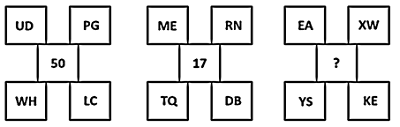Q.2 Study the diagram given below carefully and answer the question.Who among the following is an educated male who is not an urban resident?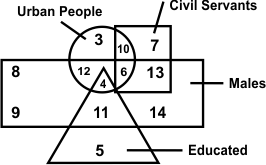Q.3 Find the missing number.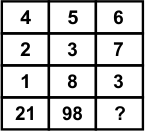Q.4 Read the following diagram carefully and answer the question:Who among the following is only a graduate?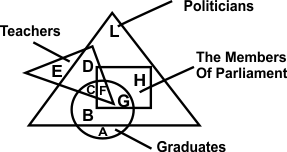Q.5 Select the correct alternative which represents the water image of the given question figure.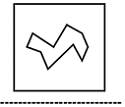Q.6 How many triangles are there in the following figure?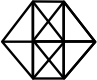Q.7 Choose the odd one out.
 Q.8 If the given figure is folded then which of the following cube is formed?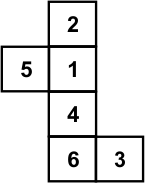Q.9 Select the number that can replace the question mark ‘?’ in the following series:24, 30, 36, 42, 52, 60, ?
 Q.10 Find the missing figure in the figure matrix.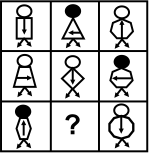Sample PDF of CREST IQ Challenge for Class 6: